# RS Aggarwal Solutions for Class 7 Maths Exercise 4E Chapter 4 Rational Numbers

RS Aggarwal Solutions for Class 7 Maths Exercise 4E Chapter 4 Rational Numbers is the best study material for those students who are finding complications in solving problems. This exercise contains multiplication of rational numbers. It is the product of two rational numbers that equals the product of their numerators by product of their denominators. Students who wish to score good marks in Maths practise RS Aggarwal Solutions for Class 7 Maths Chapter 4.

## Download the PDF of RS Aggarwal Solutions For Class 7 Maths Chapter 4 Rational Numbers – Exercise 4E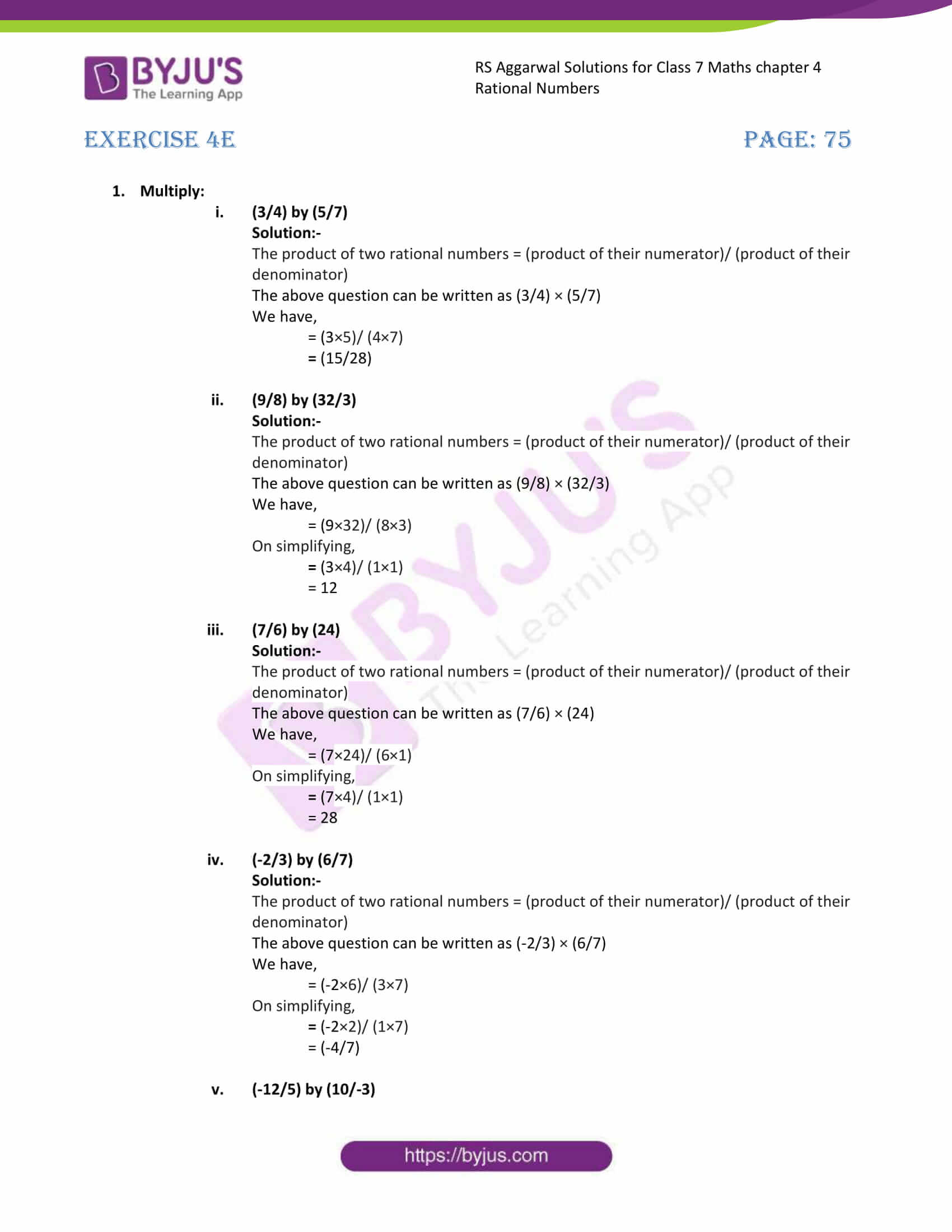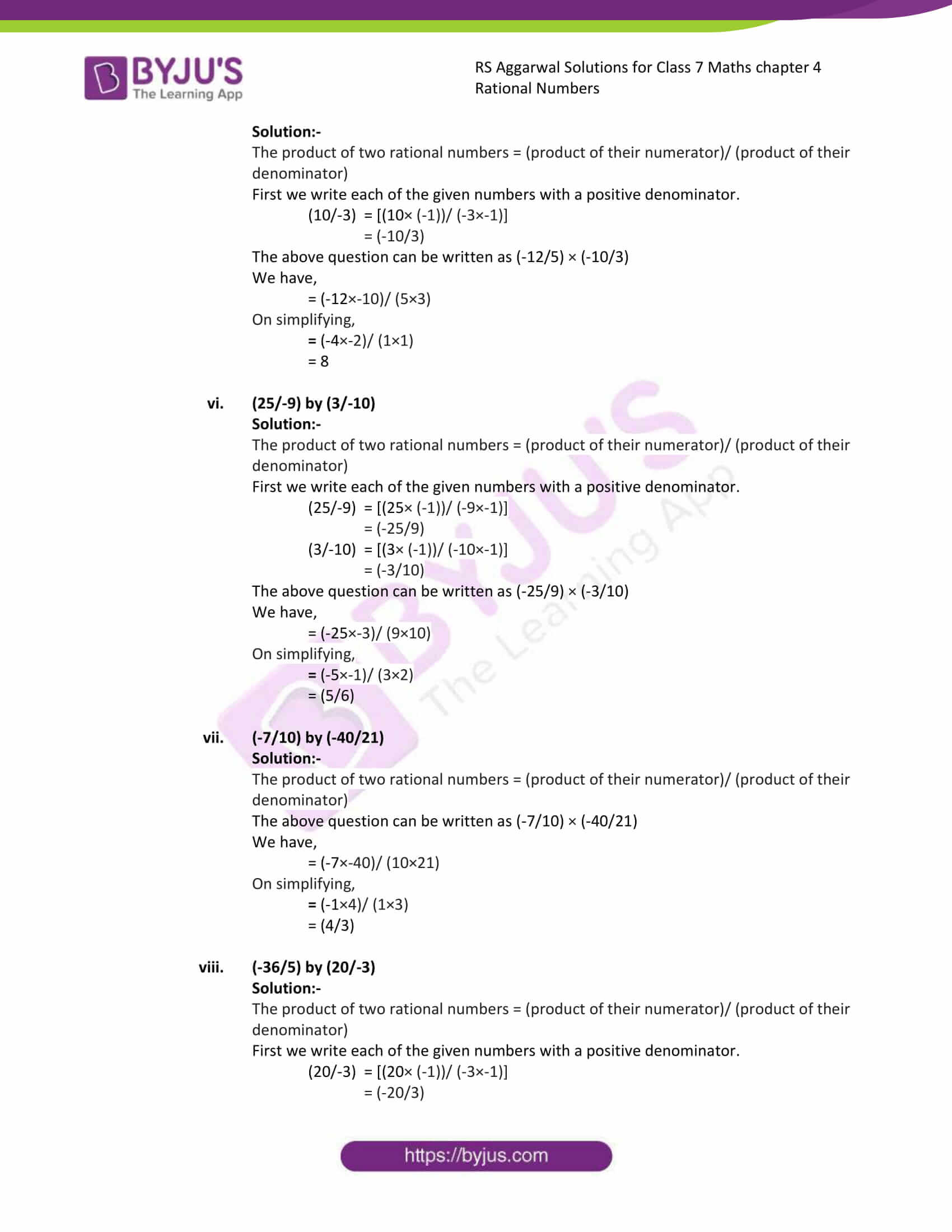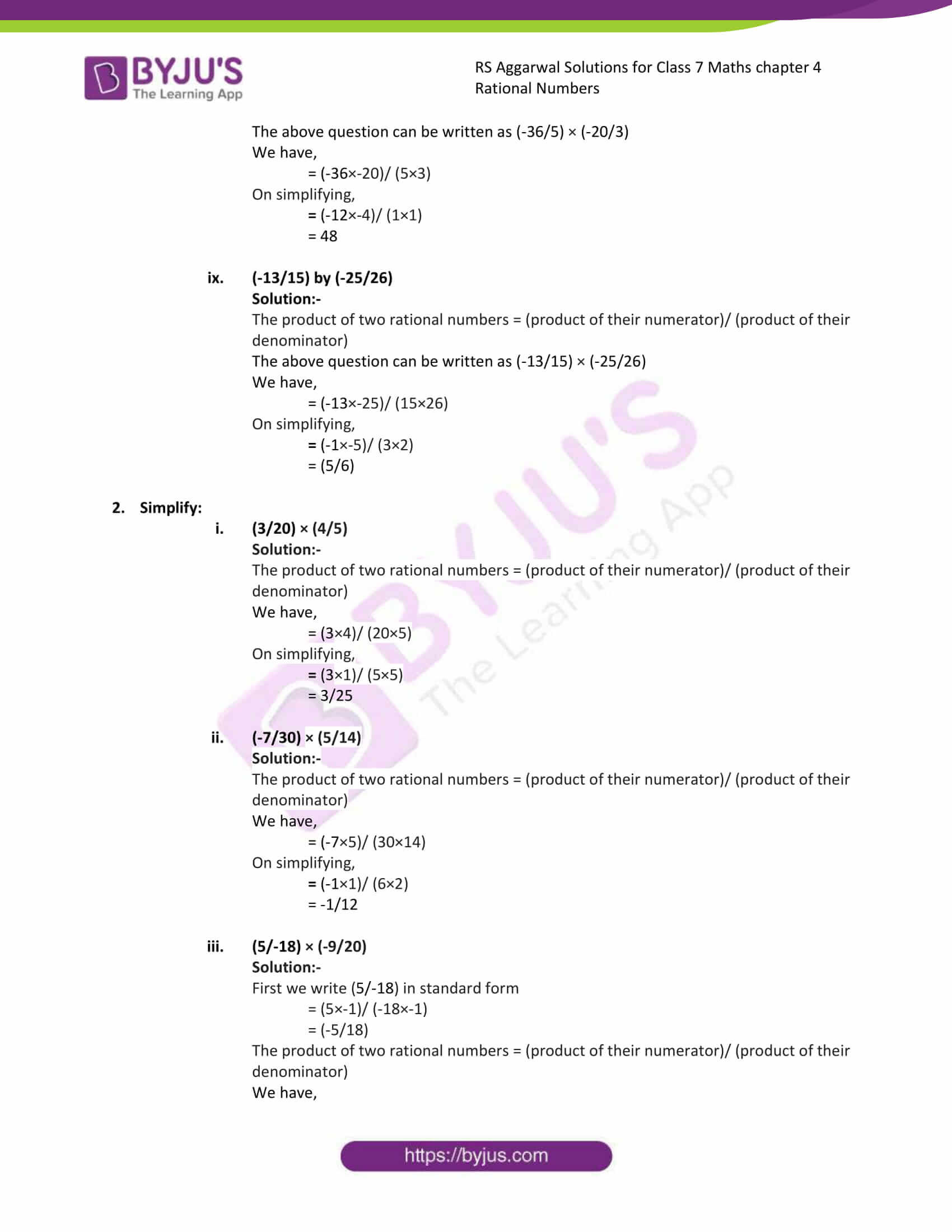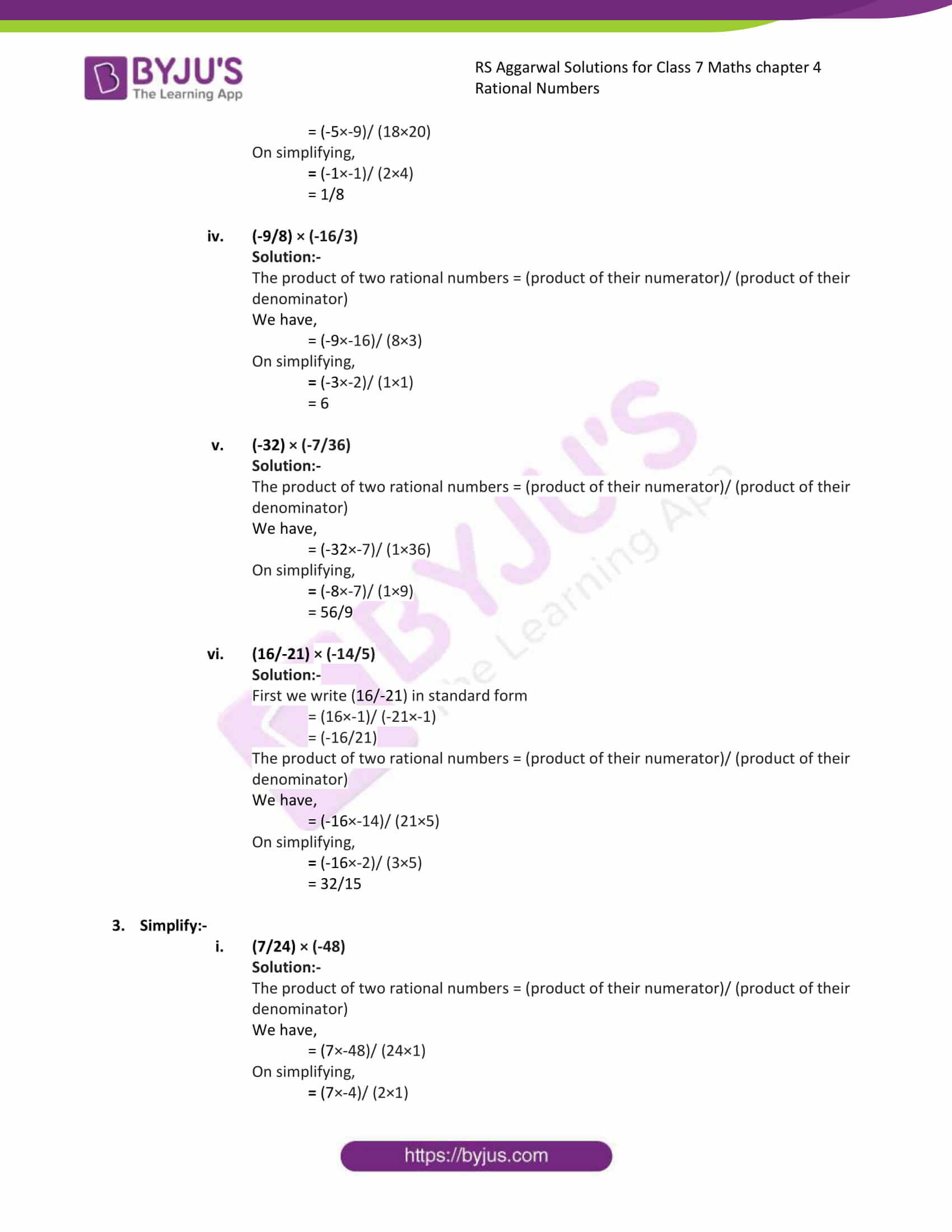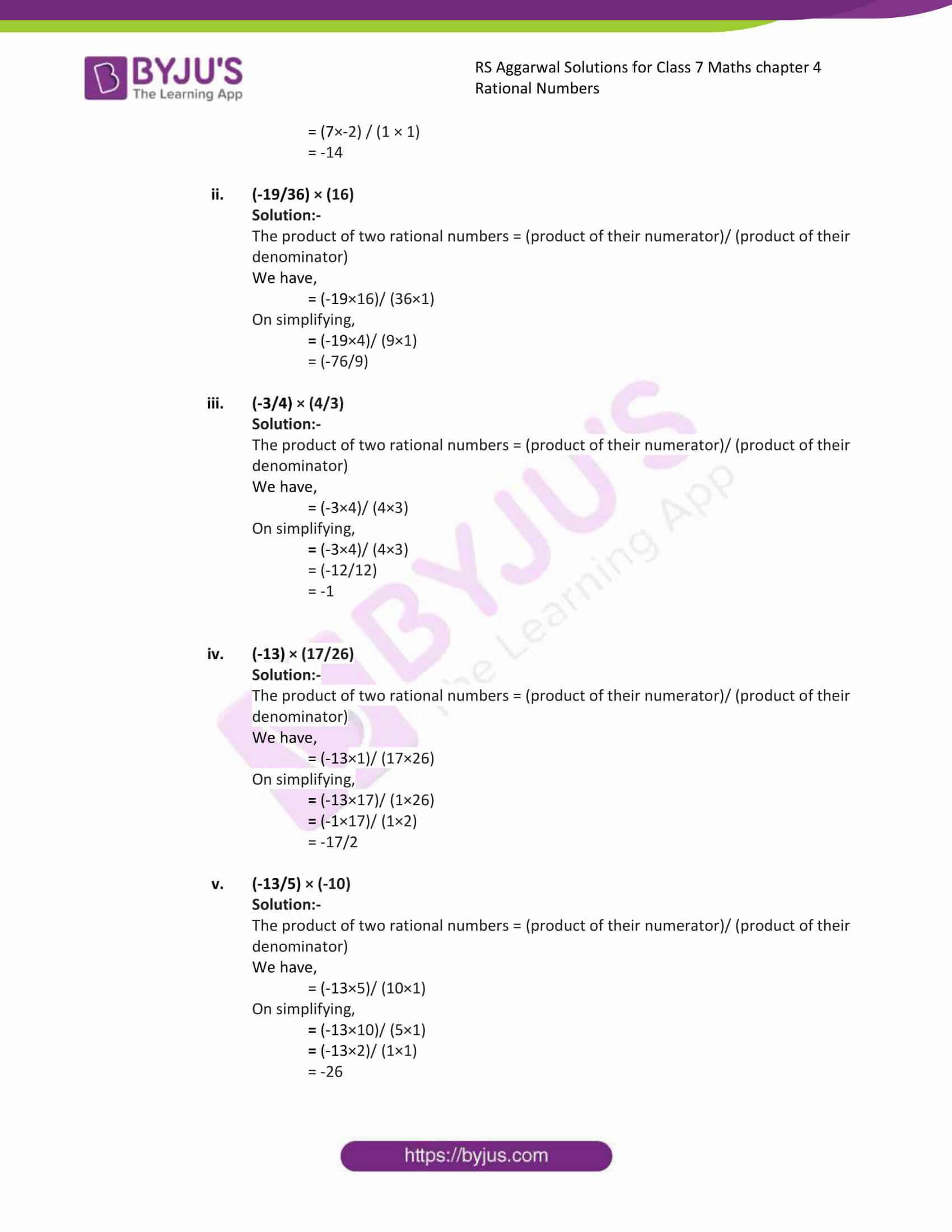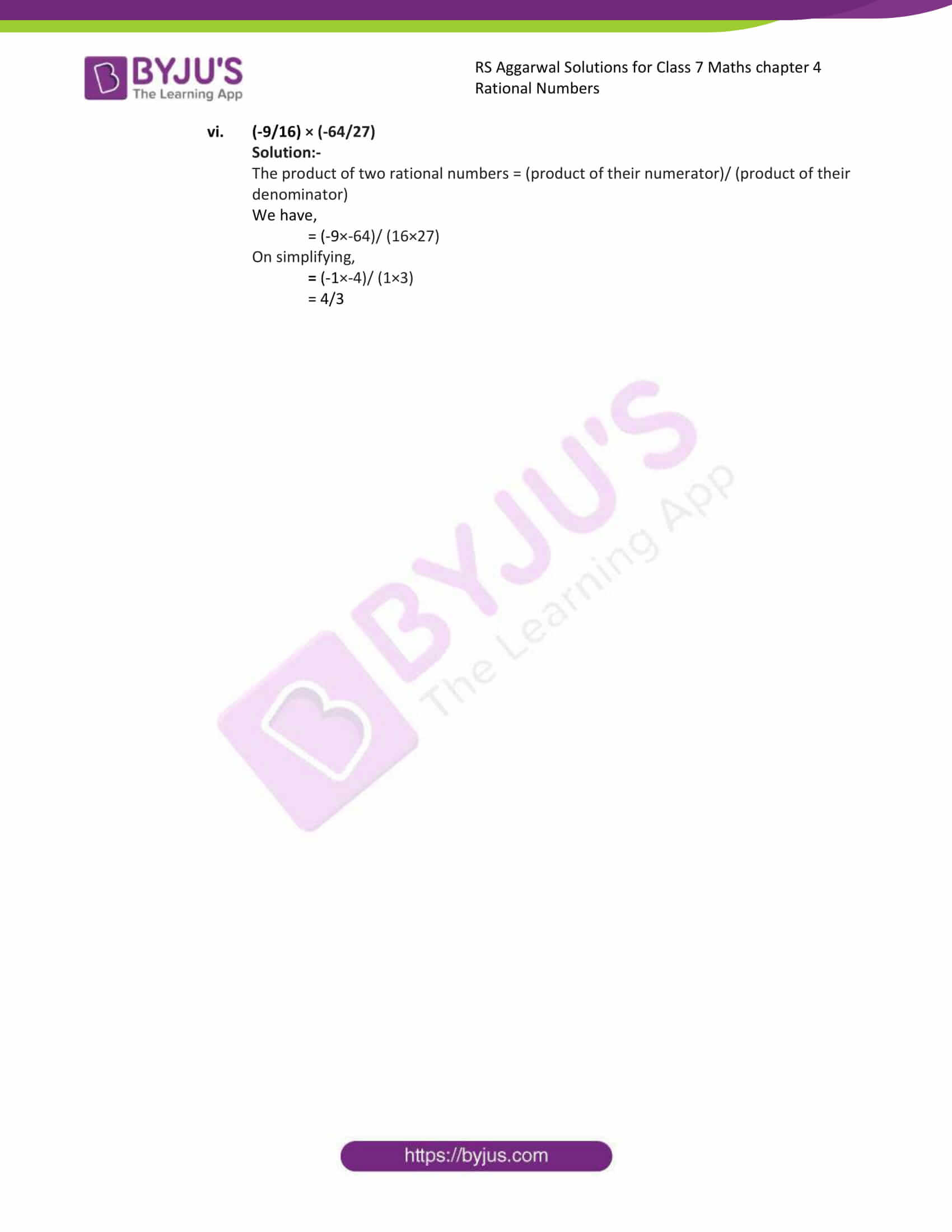### Access answers to Maths RS Aggarwal Solutions for Class 7 Chapter 4 – Rational Numbers Exercise 4E

1. Multiply:
(i) (3/4) by (5/7)

Solution:-

The product of two rational numbers = (product of their numerator)/ (product of their denominator)

The above question can be written as (3/4) × (5/7)

We have,

= (3×5)/ (4×7)

= (15/28)

(ii) (9/8) by (32/3)

Solution:-

The product of two rational numbers = (product of their numerator)/ (product of their denominator)

The above question can be written as (9/8) × (32/3)

We have,

= (9×32)/ (8×3)

On simplifying,

= (3×4)/ (1×1)

= 12

(iii) (7/6) by (24)

Solution:-

The product of two rational numbers = (product of their numerator)/ (product of their denominator)

The above question can be written as (7/6) × (24)

We have,

= (7×24)/ (6×1)

On simplifying,

= (7×4)/ (1×1)

= 28

(iv) (-2/3) by (6/7)

Solution:-

The product of two rational numbers = (product of their numerator)/ (product of their denominator)

The above question can be written as (-2/3) × (6/7)

We have,

= (-2×6)/ (3×7)

On simplifying,

= (-2×2)/ (1×7)

= (-4/7)

(v) (-12/5) by (10/-3)

Solution:-

The product of two rational numbers = (product of their numerator)/ (product of their denominator)

First we write each of the given numbers with a positive denominator.

(10/-3) = [(10× (-1))/ (-3×-1)]

= (-10/3)

The above question can be written as (-12/5) × (-10/3)

We have,

= (-12×-10)/ (5×3)

On simplifying,

= (-4×-2)/ (1×1)

= 8

(vi) (25/-9) by (3/-10)

Solution:-

The product of two rational numbers = (product of their numerator)/ (product of their denominator)

First we write each of the given numbers with a positive denominator.

(25/-9) = [(25× (-1))/ (-9×-1)]

= (-25/9)

(3/-10) = [(3× (-1))/ (-10×-1)]

= (-3/10)

The above question can be written as (-25/9) × (-3/10)

We have,

= (-25×-3)/ (9×10)

On simplifying,

= (-5×-1)/ (3×2)

= (5/6)

(vii) (-7/10) by (-40/21)

Solution:-

The product of two rational numbers = (product of their numerator)/ (product of their denominator)

The above question can be written as (-7/10) × (-40/21)

We have,

= (-7×-40)/ (10×21)

On simplifying,

= (-1×4)/ (1×3)

= (4/3)

(viii) (-36/5) by (20/-3)

Solution:-

The product of two rational numbers = (product of their numerator)/ (product of their denominator)

First we write each of the given numbers with a positive denominator.

(20/-3) = [(20× (-1))/ (-3×-1)]

= (-20/3)

The above question can be written as (-36/5) × (-20/3)

We have,

= (-36×-20)/ (5×3)

On simplifying,

= (-12×-4)/ (1×1)

= 48

(ix) (-13/15) by (-25/26)

Solution:-

The product of two rational numbers = (product of their numerator)/ (product of their denominator)

The above question can be written as (-13/15) × (-25/26)

We have,

= (-13×-25)/ (15×26)

On simplifying,

= (-1×-5)/ (3×2)

= (5/6)

2. Simplify:
(i) (3/20) × (4/5)

Solution:-

The product of two rational numbers = (product of their numerator)/ (product of their denominator)

We have,

= (3×4)/ (20×5)

On simplifying,

= (3×1)/ (5×5)

= 3/25

(ii) (-7/30) × (5/14)

Solution:-

The product of two rational numbers = (product of their numerator)/ (product of their denominator)

We have,

= (-7×5)/ (30×14)

On simplifying,

= (-1×1)/ (6×2)

= -1/12

(iii) (5/-18) × (-9/20)

Solution:-

First we write (5/-18) in standard form

= (5×-1)/ (-18×-1)

= (-5/18)

The product of two rational numbers = (product of their numerator)/ (product of their denominator)

We have,

= (-5×-9)/ (18×20)

On simplifying,

= (-1×-1)/ (2×4)

= 1/8

(iv) (-9/8) × (-16/3)

Solution:-

The product of two rational numbers = (product of their numerator)/ (product of their denominator)

We have,

= (-9×-16)/ (8×3)

On simplifying,

= (-3×-2)/ (1×1)

= 6

(v) (-32) × (-7/36)

Solution:-

The product of two rational numbers = (product of their numerator)/ (product of their denominator)

We have,

= (-32×-7)/ (1×36)

On simplifying,

= (-8×-7)/ (1×9)

= 56/9

(vi) (16/-21) × (-14/5)

Solution:-

First we write (16/-21) in standard form

= (16×-1)/ (-21×-1)

= (-16/21)

The product of two rational numbers = (product of their numerator)/ (product of their denominator)

We have,

= (-16×-14)/ (21×5)

On simplifying,

= (-16×-2)/ (3×5)

= 32/15

3. Simplify:-
(7/24) × (-48)

(i) Solution:-

The product of two rational numbers = (product of their numerator)/ (product of their denominator)

We have,

= (7×-48)/ (24×1)

On simplifying,

= (7×-4)/ (2×1)

= (7×-2) / (1 × 1)

= -14

(ii) (-19/36) × (16)

Solution:-

The product of two rational numbers = (product of their numerator)/ (product of their denominator)

We have,

= (-19×16)/ (36×1)

On simplifying,

= (-19×4)/ (9×1)

= (-76/9)

(iii) (-3/4) × (4/3)

Solution:-

The product of two rational numbers = (product of their numerator)/ (product of their denominator)

We have,

= (-3×4)/ (4×3)

On simplifying,

= (-3×4)/ (4×3)

= (-12/12)

= -1

(iv) (-13) × (17/26)

Solution:-

The product of two rational numbers = (product of their numerator)/ (product of their denominator)

We have,

= (-13×1)/ (17×26)

On simplifying,

= (-13×17)/ (1×26)

= (-1×17)/ (1×2)

= -17/2

(v) (-13/5) × (-10)

Solution:-

The product of two rational numbers = (product of their numerator)/ (product of their denominator)

We have,

= (-13×5)/ (10×1)

On simplifying,

= (-13×10)/ (5×1)

= (-13×2)/ (1×1)

= -26

(vi) (-9/16) × (-64/27)

Solution:-

The product of two rational numbers = (product of their numerator)/ (product of their denominator)

We have,

= (-9×-64)/ (16×27)

On simplifying,

= (-1×-4)/ (1×3)

= 4/3

### Access other exercises of RS Aggarwal Solutions For Class 7 Chapter 4 – Rational Numbers

Exercise 4G Solutions